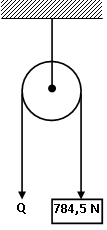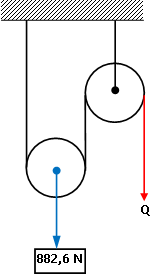Static

# Guide n° 2 of exercises of statics

## Solve the following exercises

### problem #1

Two 10-N weights are suspended at the ends of a rope that passes over a light, frictionless pulley. The pulley is attached to a chain that hangs from the ceiling. Decide:

a) The tension in the string.

b) The chain tension.

## Statement of exercise n° 1

Two 10-N weights are suspended at the ends of a rope that passes over a light, frictionless pulley. The pulley is attached to a chain that hangs from the ceiling. Decide:

a) The tension in the string.

b) The chain tension.

### Developing

P1 = P2 10N

#### Formulas:

P = m g

∑F = 0 (equilibrium condition)

Equilibrium condition: The sum of the moments of the forces must be zero: Newton’s first law (equilibrium)

### Solution

First we make a scheme to correctly visualize the statement:to)

We make the free body diagram for one of the strings:We set the equilibrium conditions:

∑F = 0

P1 – T1 = 0

We clear T 1 :

T1 = P1 _

We replace by the value of P:

T1 = 10N

The same result will be obtained for T 2 :

Result, string tension is:

T1 = T2 10N

b)

We draw the free body diagram for the chain:We set the equilibrium conditions:

∑F = 0

P – T = 0

In this case the weight P is the sum of the weights hanging from the strings:

P = P1 + P2

P = 10N + 10N

P = 20N

We clear the tension T:

T = P

Result, chain tension is:

T = 20N

### problem #2

In a mechanical workshop, the engine of a car, whose weight is 350 kgf, is lifted by means of a differential hoist. If the radii of the pulleys are R = 15 cm and r = 12 cm, what is the force that balances that weight?

## Statement of exercise n° 2

In a mechanical workshop, the engine of a car, whose weight is 350 kgf, is lifted by means of a differential hoist. If the radii of the pulleys are R = 15 cm and r = 12 cm, what is the force that balances that weight?

### Developing

#### Data:

P = 350 kgf = 3,432.3275 N

R=15cm=0.15m

r = 12cm = 0.12m

#### Formulas:

 F = R – r ·P 2 R### Solution

We directly apply the differential rigging formula, replacing it with the data after converting the units:

 F = 0.15m-0.12m 3,432.3275N 2 0.15m
 F = 0.03m 3,432.3275N 0.3m

F = 343.23275N

Result, the force that balances that weight of the motor is:

F = 343.2N

### Problem #3

The radii of a differential rig are R = 20 cm and r = 15 cm. If a force of 80 kgf is applied, what is the weight of the body that balances it?

## Statement of exercise n° 3

The radii of a differential rig are R = 20 cm and r = 15 cm. If a force of 80 kgf is applied, what is the weight of the body that balances it?

### Developing

#### Data:

F = 80 kgf = 784.532 N

R=20cm=0.2m

r = 15cm = 0.15m

#### Formulas:

 F = R – r ·P 2 R

#### Scheme:### Solution

We clear the weight of the differential rigging formula:

 P = F 2 R R – r

We replace by the data after converting the units:

 P = 313.8128 N m 0.05m

P = 6,276.256N

Result, the body weight is:

P = 6,276.3N

### Problem #4

In a 2nd gender lever, it has a power arm Bp = 1.20 m and a resistance arm Br = 8 cm. Then the multiplication of the lever is:

a) 6

b) 12

c) 15

c) 20

## Statement of exercise n° 4

In a 2nd gender lever, it has a power arm Bp = 1.20 m and a resistance arm Br = 8 cm. Then the multiplication of the lever is:

a) 6

b) 12

c) 15

c) 20

### Developing

Bp = 1.20m

Br = 8cm = 0.08m

#### Formulas:

 M = bp br

### Solution

Without calculating the moments, the multiplication ratio is always the power arm over the resistance arm, so:

 M = 1.20m 0.08m

Result, the multiplication of the lever is:

M = 15

### Problem #5

Calculate at what distance from a 60 kgf power a rigid iron bar will be supported, to balance a 300 kgf box that is 0.75 m from the support.

## Statement of exercise n° 5

Calculate at what distance from a 60 kgf power a rigid iron bar will be supported, to balance a 300 kgf box that is 0.75 m from the support.

### Developing

#### Data:

F = 60 kgf = 588.399 N

P = 300 kgf = 2,941.995 N

BP = 0.75m

#### Formulas:

MF = 0 (Equilibrium condition of moments)

F B F = P B P

### Solution

It is understood that it is a 1st class lever.

From the lever equation we isolate the arm of the force (B F ):

 B F = P B P F

We substitute for the data and calculate:

 B F = 2,941.995N 0.75m 588,399N
 B F = 2,206.49625m 588,399

Result, the distance between the stem and the support point is:

BF = 3.75m

### Problem #6

Calculate the power that is necessary to apply to a fixed pulley, to lift a weight of 80 kgf.

## Statement of exercise n° 6

Calculate the power that is necessary to apply to a fixed pulley, to lift a weight of 80 kgf.

### Developing

#### Data:

P = 80 kgf = 784.5 N

#### Formulas:

∑F = 0 (equilibrium condition)

Equilibrium condition: The sum of the moments of the forces must be zero: Newton’s first law (equilibrium)

### Solution

First we make a scheme to correctly visualize the statement:We apply the equilibrium condition taking into account that in the fixed pulley the weight and the power are equal and opposite forces:

∑F = 0

P – Q = 0

Q=P

Result, the power applied to the pulley is:

Q = 784.5N

### Problem #7

What power will be applied to balance a resistance of 90 kgf, by means of a mobile pulley?

Solution to problem #7

## Statement of exercise No. 7

What power will be applied to balance a resistance of 90 kgf, by means of a mobile pulley?

### Developing

#### Data:

P = 90 kgf = 882.6 N

Q = ½ P

### Solution

We make the scheme:We apply the simple mobile pulley formula:

Q = ½ P

We substitute and calculate:

Q = ½ 882.6 N

Result, the power applied to the pulley is:

Q = 441.3N

### Problem #8

A rope is wrapped around a cylinder with a diameter of 30 cm (which can rotate around an axis). If a force (weight) of 1.8 kgf is applied to it, what is the value of the moment that rotates the cylinder?

## Statement of exercise n° 8

A rope is wrapped around a cylinder with a diameter of 30 cm (which can rotate around an axis). If a force (weight) of 1.8 kgf is applied to it, what is the value of the moment that rotates the cylinder?

### Developing

#### Data:

P = 1.8 kgf = 17.65197 N

d = 30cm = 0.3m

M P = P r

### Solution

We know that:

d = 2 r

r = ½ d

r = ½ 0.3m

r = 0.15m

We apply the formula for now:

M P = P r

We substitute for the data and calculate:

M P = 17.65197 N 0.15 m

M P = 2.6477955 N m

Result, the moment produced by the weight that rotates the cylinder is:

M P = 2.65 N m